# Pandas Built-in Data Visualization | ML

Data Visualization is the presentation of data in graphical format. It helps people understand the significance of data by summarizing and presenting a huge amount of data in a simple and easy-to-understand format and helps communicate information clearly and effectively.

In this tutorial, we will learn about pandas built-in capabilities for data visualization! It’s built-off of matplotlib, but it baked into pandas for easier usage!

Let’s take a look!

Installation
Easiest way to install pandas is to use pip:

```pip install pandas
```

This article demonstrates an illustration of using built-in data visualization feature in pandas by plotting different types of charts.

### Importing necessary libraries and data files –

The Sample csv files df1 and df2 used in this tutorial can be downloaded from here.

 `import` `numpy as np ` `import` `pandas as pd ` ` `  `# There are some fake data csv files ` `# you can read in as dataframes ` `df1 ``=` `pd.read_csv(``'df1'``, index_col ``=` `0``) ` `df2 ``=` `pd.read_csv(``'df2'``) `

### Style Sheets –

Matplotlib has style sheets which can be used to make the plots look a little nicer. These style sheets include `plot_bmh`, `plot_fivethirtyeight`, `plot_ggplot `and more. They basically create a set of style rules that your plots follow. We recommend using them, they make all your plots have the same look and feel more professional. We can even create our own if want company’s plots to all have the same look (it is a bit tedious to create on though).

Here is how to use them.

Before` plt.style.use()` plots look like this:

 `df1[``'A'``].hist() `

Output :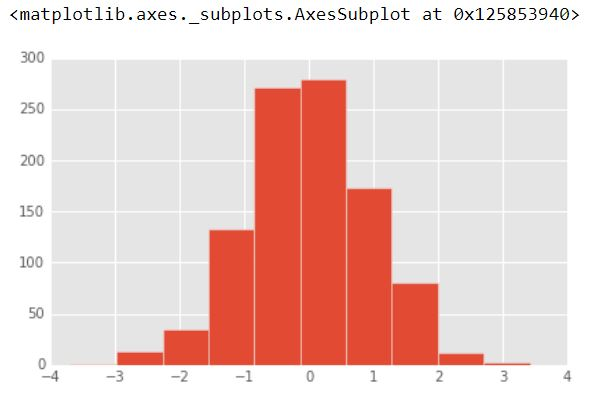Call the style:

Now, plots look like this after calling `ggplot` style:

 `import` `matplotlib.pyplot as plt ` `plt.style.use(``'ggplot'``) ` `df1[``'A'``].hist() `

Output :Plots look like this after calling `bmh` style:

 `plt.style.use(``'bmh'``) ` `df1[``'A'``].hist() `

Output :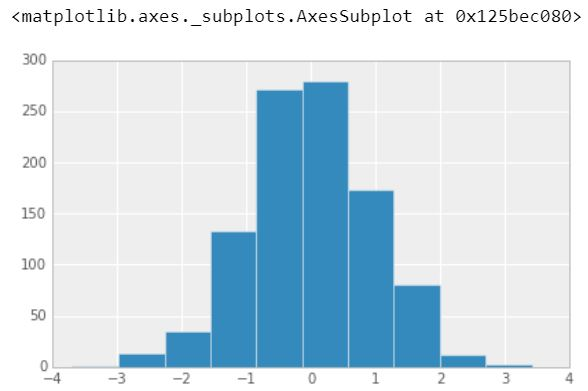Plots look like this after calling `dark_background` style:

 `plt.style.use(``'dark_background'``) ` `df1[``'A'``].hist() `

Output :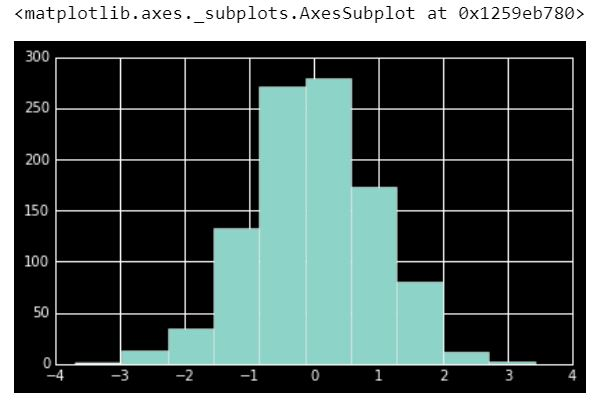Plots look like this after calling `fivethirtyeight` style:

 `plt.style.use(``'fivethirtyeight'``) ` `df1[``'A'``].hist() `

Output :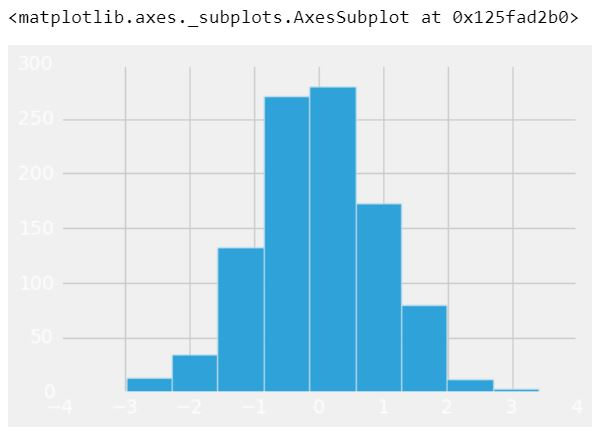### Plot Types –

There are several plot types built-in to pandas, most of them statistical plots by nature:

• df.plot.area
• df.plot.barh
• df.plot.density
• df.plot.hist
• df.plot.line
• df.plot.scatter
• df.plot.bar
• df.plot.box
• df.plot.hexbin
• df.plot.kde
• df.plot.pie
• You can also just call `df.plot(kind='hist') `or replace that kind argument with any of the key terms shown in the list above (e.g. ‘box’, ‘barh’, etc.). Let’s start going through them!

### 1.) Area

An area chart or area graph displays graphically quantitative data. It is based on the line chart. The area between axis and line are commonly emphasized with colors, textures and hatchings. Commonly one compares two or more quantities with an area chart.

 `df2.plot.area(alpha ``=` `0.4``) `

Output :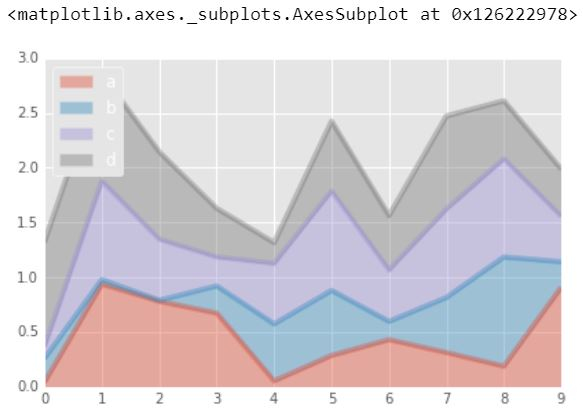### 2.) Barplots

A bar chart or bar graph is a chart or graph that presents categorical data with rectangular bars with heights or lengths proportional to the values that they represent. The bars can be plotted vertically or horizontally. A vertical bar chart is sometimes called a line graph.

 `df2.head() `

Output :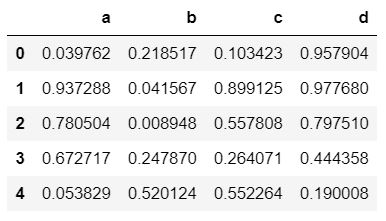`df2.plot.bar() `

Output :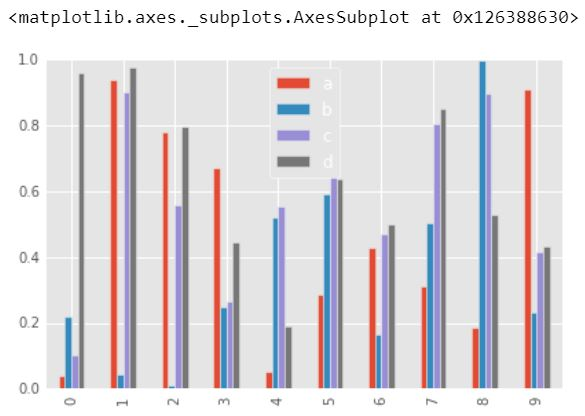`df2.plot.bar(stacked ``=` `True``) `

Output :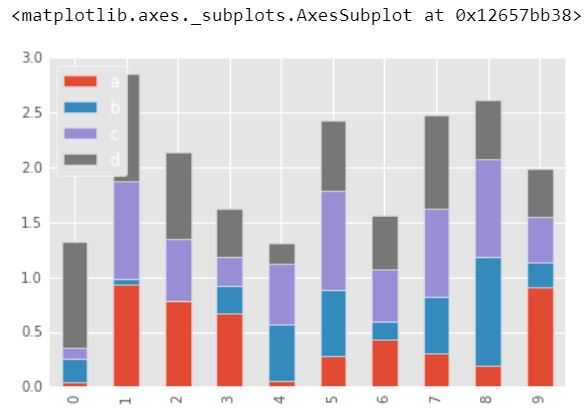### 3.) Histograms

A histogram is a plot that lets you discover, and show, the underlying frequency distribution (shape) of a set of continuous data. This allows the inspection of the data for its underlying distribution (e.g., normal distribution), outliers, skewness, etc.

 `df1[``'A'``].plot.hist(bins ``=` `50``) `

Output :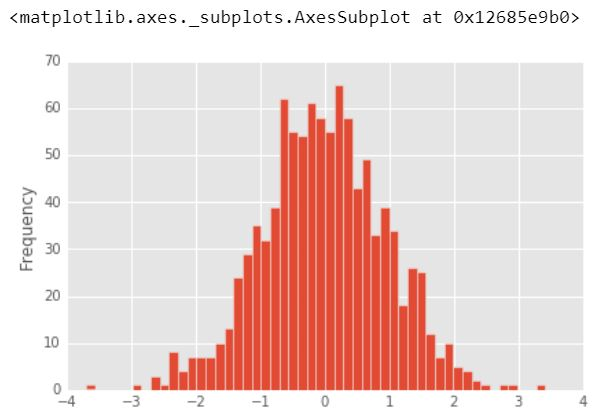### 4.) Line Plots

A line plot is a graph that shows frequency of data along a number line. It is best to use a line plot when the data is time series. It is a quick, simple way to organize data.

 `df1.plot.line(x ``=` `df1.index, y ``=``'B'``, figsize ``=``(``12``, ``3``), lw ``=` `1``) `

Output :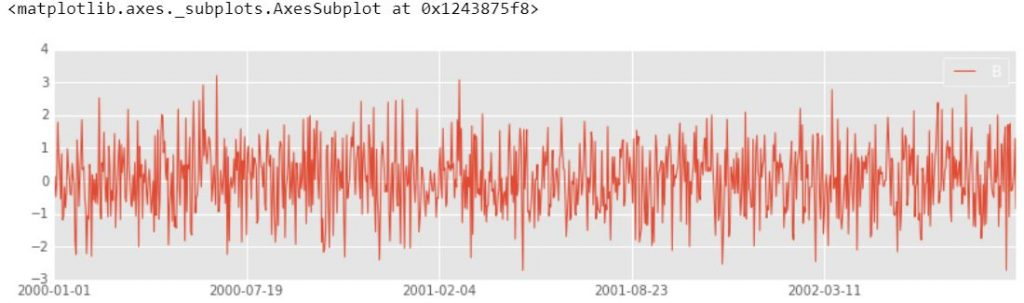### 5.) Scatter Plots

Scatter plots are used when you want to show the relationship between two variables. Scatter plots are sometimes called correlation plots because they show how two variables are correlated.

 `df1.plot.scatter(x ``=``'A'``, y ``=``'B'``) `

Output :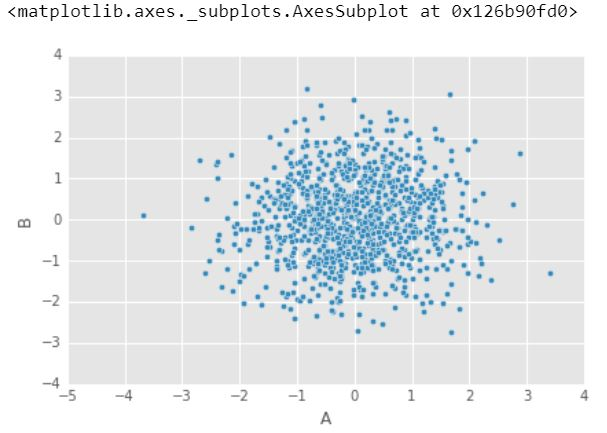You can use c to color based off another column value Use cmap to indicate colormap to use. For all the colormaps, check out: http://matplotlib.org/users/colormaps.html

 `df1.plot.scatter(x ``=``'A'``, y ``=``'B'``, c ``=``'C'``, cmap ``=``'coolwarm'``) `

Output :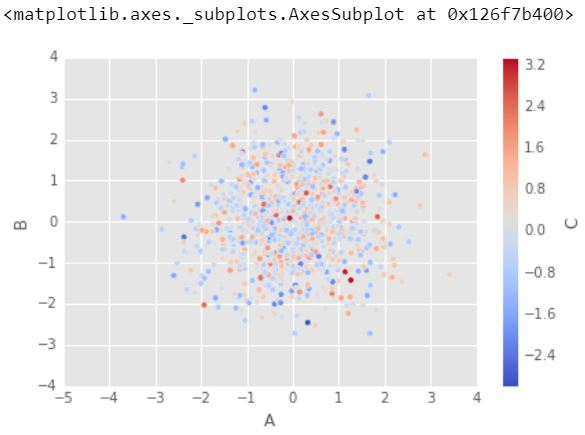Or use s to indicate size based off another column. s parameter needs to be an array, not just the name of a column:

 `df1.plot.scatter(x ``=``'A'``, y ``=``'B'``, s ``=` `df1[``'C'``]``*``200``) `

Output :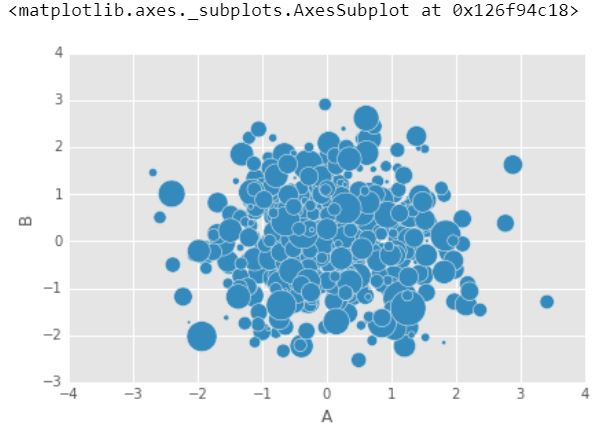### 6.) Box Plots

It is a plot in which a rectangle is drawn to represent the second and third quartiles, usually with a vertical line inside to indicate the median value. The lower and upper quartiles are shown as horizontal lines either side of the rectangle.
A boxplot is a standardized way of displaying the distribution of data based on a five number summary (“minimum”, first quartile (Q1), median, third quartile (Q3), and “maximum”). It can tell you about your outliers and what their values are. It can also tell you if your data is symmetrical, how tightly your data is grouped, and if and how your data is skewed.

 `df2.plot.box() ``# Can also pass a by = argument for groupby `

Output :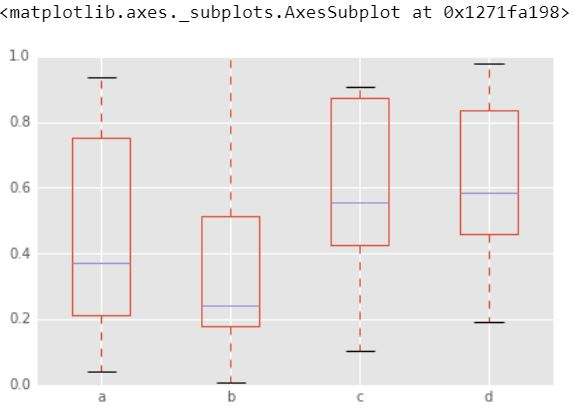### 7.) Hexagonal Bin Plots

Hexagonal Binning is another way to manage the problem of having to many points that start to overlap. Hexagonal binning plots density, rather than points. Points are binned into gridded hexagons and distribution (the number of points per hexagon) is displayed using either the color or the area of the hexagons.
Useful for Bivariate Data, alternative to scatterplot:

 `df ``=` `pd.DataFrame(np.random.randn(``1000``, ``2``), columns ``=``[``'a'``, ``'b'``]) ` `df.plot.hexbin(x ``=``'a'``, y ``=``'b'``, gridsize ``=` `25``, cmap ``=``'Oranges'``) `

Output :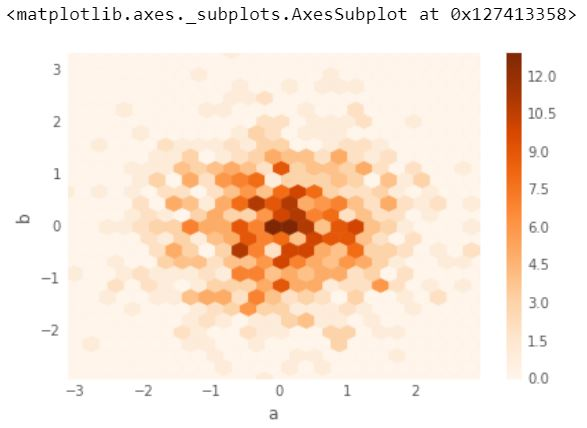### 8.) Kernel Density Estimation plot (KDE)

KDE is a technique that let’s you create a smooth curve given a set of data.

This can be useful if you want to visualize just the “shape” of some data, as a kind of continuous replacement for the discrete histogram. It can also be used to generate points that look like they came from a certain dataset – this behavior can power simple simulations, where simulated objects are modeled off of real data.

 `df2[``'a'``].plot.kde() `

Output :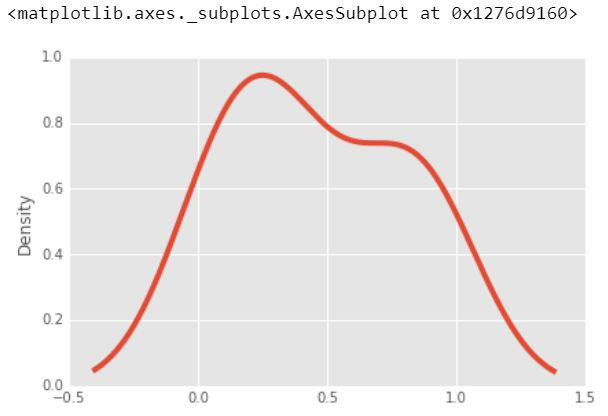`df2.plot.density() `

Output :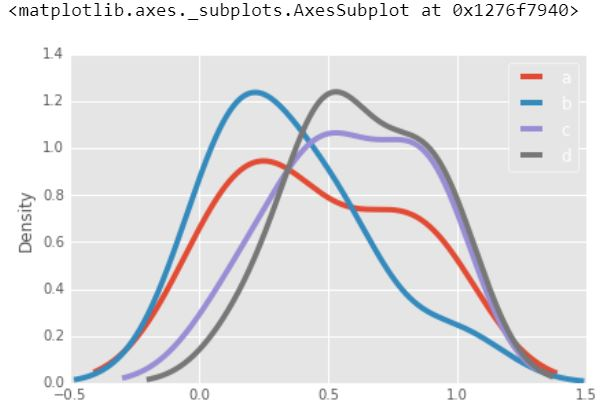That’s it! Hopefully you can see why this method of plotting will be a lot easier to use than full-on matplotlib, it balances ease of use with control over the figure. A lot of the plot calls also accept additional arguments of their parent matplotlib plt. call.

My Personal Notes arrow_drop_upCheck out this Author's contributed articles.

If you like GeeksforGeeks and would like to contribute, you can also write an article using contribute.geeksforgeeks.org or mail your article to contribute@geeksforgeeks.org. See your article appearing on the GeeksforGeeks main page and help other Geeks.

Please Improve this article if you find anything incorrect by clicking on the "Improve Article" button below.